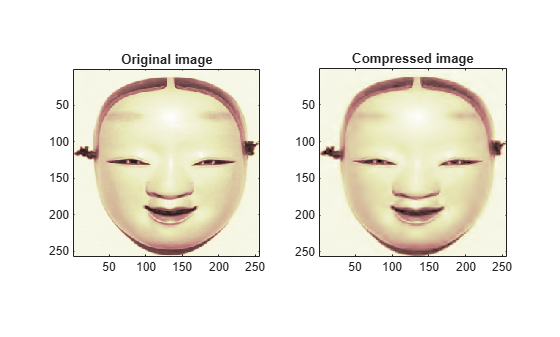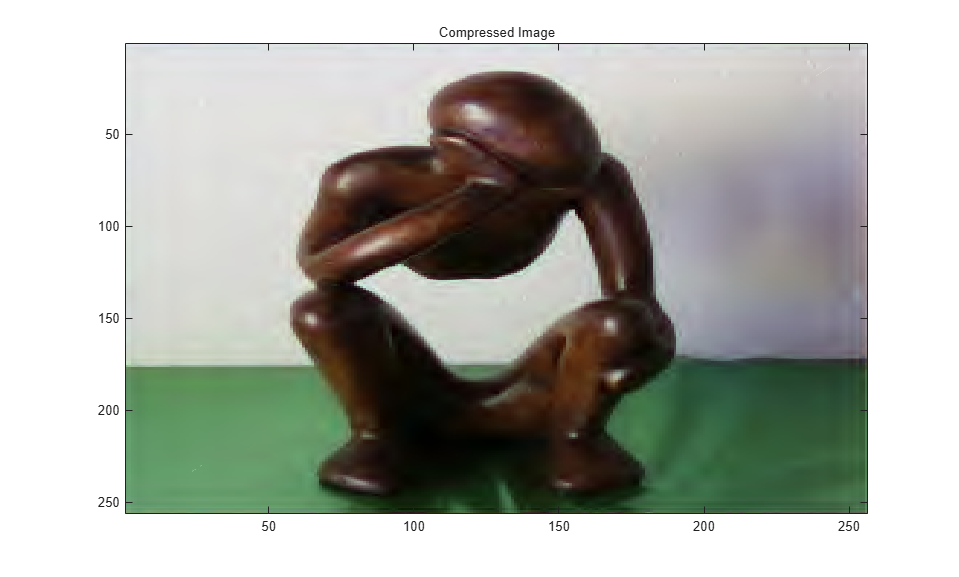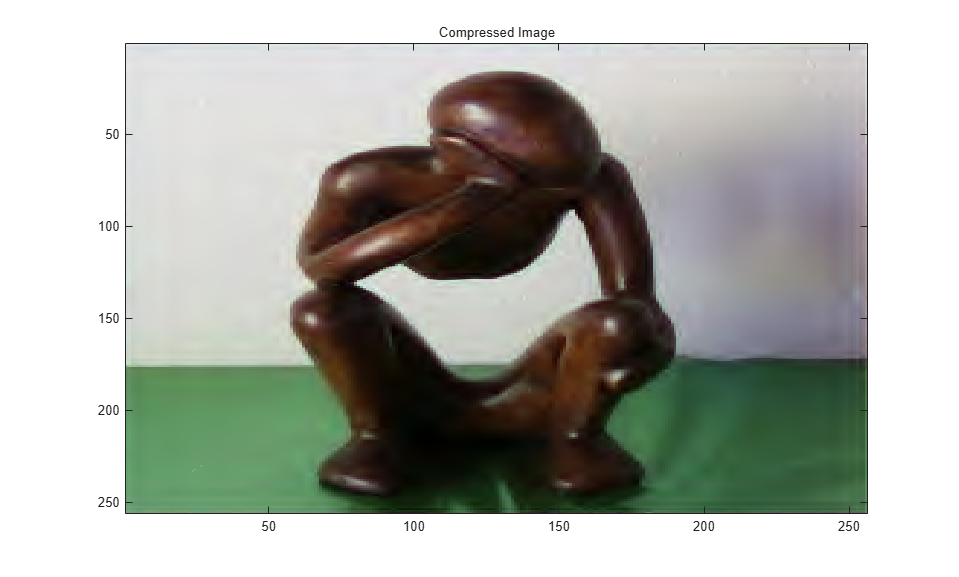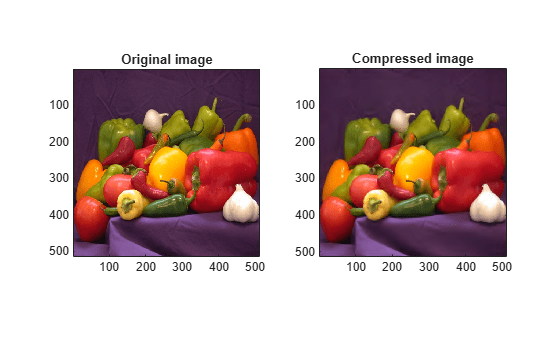# wcompress

True compression of images using wavelets

## Syntax

``wcompress('c',x,cname,compmthd)``
``wcompress('c',fname,___)``
``wcompress('c',I,___)``
``wcompress(___,Name,Value)``
``[comprat,bpp] = wcompress('c',___)``
``xc = wcompress('u',cname)``
``xc = wcompress('u',cname,'plot')``
``xc = wcompress('u',cname,'step')``

## Description

The `wcompress` function performs either compression or uncompression of grayscale or truecolor images.

### Compression

````wcompress('c',x,cname,compmthd)` compresses the image `x` using the compression method `compmthd` and saves the result in the file `cname`. The image `x` can be either a 2-D array containing an indexed image or a 3-D array of `uint8` containing a truecolor image. Both the row and column size of the image must be powers of two.You must have write permission in the current working directory or the function will change directory to `tempdir` and write the compressed image in that directory. NoteThe Discrete Wavelet Transform uses the periodized extension mode.Data written to the files uses `uint64` precision. In releases previous to R2016b, data was written using `uint32` . If your code is affected adversely by this change, use the `legacy` option to compress and uncompress your data using the previous behavior.wcompress('c',x,cname,compmthd,'legacy') ```

example

````wcompress('c',fname,___)` loads the image from the file `fname`.```
````wcompress('c',I,___)` converts the indexed image `I{1}` to a truecolor image Y using the colormap `I{2}` and then compresses Y.```

example

````wcompress(___,Name,Value)` specifies options related to display, data transform, and compression methods using one or more name-value pair arguments in addition to the input arguments in previous syntaxes. The name can be in uppercase or lowercase. For example, `'level',3,'CC','klt'` sets the level of the decomposition to 3 and the Color Conversion parameter if `x` is a truecolor image to the Karhunen-Loève transform.```
````[comprat,bpp] = wcompress('c',___)` returns the compression ratio `comprat` and the bit-per-pixel ratio `bpp`.```

### Uncompression

````xc = wcompress('u',cname)` uncompresses the file `cname` which contains the compressed image, and the returns the image `xc`.```
````xc = wcompress('u',cname,'plot')` plots the uncompressed image.```

example

````xc = wcompress('u',cname,'step')` shows the step-by-step uncompression (only for Progressive Coefficients Significance Methods).```

## Examples

collapse all

This example shows how to compress and uncompress the jpeg image arms.jpg.

Use the spatial orientation tree wavelet (`'stw'`) compression method and save the compressed image to a file.

```wcompress('c','arms.jpg','comp_arms.wtc','stw'); ```

Load the stored image and display the step-by-step uncompression to produce the uncompressed image.

```wcompress('u','comp_arms.wtc','step'); ```This example shows how to compress a grayscale image using the set partitioning in hierarchical trees (`'spiht'`) compression method. It also computes the mean square error (MSE) and the peak signal to noise ratio (PSNR) error values. You use these two measures to quantify the error between two images. The PSNR is expressed in decibels.

Load the image and store it in a file.

```load mask; [cr,bpp] = wcompress('c',X,'mask.wtc','spiht','maxloop',12)```
```cr = 2.8610 ```
```bpp = 0.2289 ```

Load the stored image from the file, uncompress it, and delete the file.

```Xc = wcompress('u','mask.wtc'); delete('mask.wtc')```

Display the original and compressed images.

```colormap(pink(255)) subplot(1,2,1); image(X); title('Original image') axis square subplot(1,2,2); image(Xc); title('Compressed image') axis square```Compute the MSE and PSNR.

```D = abs(X-Xc).^2; mse = sum(D(:))/numel(X)```
```mse = 33.6564 ```
`psnr = 10*log10(255*255/mse)`
```psnr = 32.8601 ```

This example show how to compress a jpeg image using the adaptively scanned wavelet difference reduction compression method (`'aswdr'`). The conversion color (`'cc'`) uses the Karhunen-Loeve transform (`'kit'`). The maximum number of loops (`'maxloop'`) is set to 11 and the plot type (`'plotpar'`) is set to step through the compression. Show the compression ratio (`cratio`) and the bit-per-pixel ratio (`bpp`), which indicate the quality of the compression.

```[cratio,bpp] = wcompress('c','woodstatue.jpg','woodstatue.wtc', ... 'aswdr','cc','klt','maxloop',11,'plotpar','step'); cratio bpp ```
```cratio = 3.0792 bpp = 0.7390 ```Load the compressed image and step through the uncompression process.

```wcompress('u','woodstatue.wtc','step'); ```This example shows how to compress a truecolor image using the set partitioning in hierarchical trees - 3D (`'spiht_3D'`) compression method.

Load, compress, and store the image in a file. Plot the original and compressed images. Display the compression ratio (`'cratio'`) and the bits-per-pixel (`'bpp'`), which indicate the quality of the compression.

```load mask; X = imread('wpeppers.jpg'); [cratio,bpp] = wcompress('c',X,'wpeppers.wtc','spiht','maxloop',12)```
```cratio = 1.6549 ```
```bpp = 0.3972 ```
```Xc = wcompress('u','wpeppers.wtc'); delete('wpeppers.wtc')```

Display the original and compressed images.

```subplot(1,2,1) image(X) title('Original image') axis square subplot(1,2,2) image(Xc) title('Compressed image') axis square```Compute the mean square error (MSE) and the peak signal-to-noise ratio (PSNR) error values. You use these two measures to quantify the error between two images. The PSNR is expressed in decibels.

```D = abs(double(X)-double(Xc)).^2; mse = sum(D(:))/numel(X)```
```mse = 26.7808 ```
`psnr = 10*log10(255*255/mse)`
```psnr = 33.8526 ```

## Input Arguments

collapse all

Input image to compress, specified as a 2-D array containing an indexed image or a 3-D array of `uint8` containing a truecolor image. Both the row and column size of the image must be powers of two.

Data Types: `single` | `double` | `int8` | `int16` | `int32` | `int64` | `uint8` | `uint16` | `uint32` | `uint64`

#### Compression

Compressed image filename, specified as a character vector or string scalar. The `wcompress` function writes the compressed image to the file `cname`.

#### Uncompression

Compressed image filename, specified as a character vector or string scalar. The `wcompress` function reads the compressed image from the file `cname` for uncompression.

Image filename, specified as a character vector or string scalar. The file is a MATLAB® Supported Format (MSF) file: MAT-file or other image files (see `imread`).

Compression method, specified as a character vector or string scalar. The valid compression methods are divided into two categories.

• Progressive Coefficients Significance Methods (PCSM):

compmthd

Compression Method Name

`'ezw'`

Embedded Zerotree Wavelet

`'spiht'`

Set Partitioning In Hierarchical Trees

`'stw'`

Spatial-orientation Tree Wavelet

`'wdr'`

Wavelet Difference Reduction

`'aswdr'`

`'spiht_3d'`

Set Partitioning In Hierarchical Trees 3D for truecolor images

For additional information of these methods, see the references and especially  and .

• Coefficients Thresholding Methods (CTM):

compmthd

Compression Method Name

`'lvl_mmc'`

Subband thresholding of coefficients and Huffman encoding

`'gbl_mmc_f'`

Global thresholding of coefficients and fixed encoding

`'gbl_mmc_h'`

Global thresholding of coefficients and Huffman encoding

For additional details on the `'lvl_mmc'` method, see 

### Name-Value Arguments

Specify optional pairs of arguments as `Name1=Value1,...,NameN=ValueN`, where `Name` is the argument name and `Value` is the corresponding value. Name-value arguments must appear after other arguments, but the order of the pairs does not matter.

Before R2021a, use commas to separate each name and value, and enclose `Name` in quotes.

Example: `'IT','g'` sets the Image type transform to grayscale.

Data Transform Parameters

collapse all

Wavelet name, specified as the comma-separated pair consisting of `'wname'` and a character vector or string scalar. See `waveletfamilies`.

Level of wavelet decomposition, specified as the comma-separated pair consisting of `'level'` and a positive integer. The decomposition level `level` must be such that `1 ≤ level ≤ levmax`, where `levmax` is the maximum possible level (see `wmaxlev`).

The default level depends on the compression method `compmthd`.

• For PCSM methods,`level` is equal to `levmax`.

• For CTM methods, `level` is equal to `fix(levmax/2)`.

Data Types: `single` | `double`

Image type transform, specified as the comma-separated pair consisting of `'it'` and one of the values listed:

• `'n'` — no transformation (default), image type (truecolor or grayscale) is automatically detected

• `'g'` — grayscale transformation type

• `'c'` — color transformation type (RGB `uint8`)

Color conversion parameter, specified as the comma-separated pair consisting of `'cc'` and one of the values listed:

• `'rgb'` or `'none'` — no conversion (default)

• `'yuv'` — YUV color space transform

• `'klt'` — Karhunen-Loève transform

• `'yiq'` — YIQ color space transform

• `'xyz'` — CIEXYZ color space transform

Progressive Coefficients Significance Methods (PCSM)

collapse all

Maximum number of steps for the compression algorithm, specified as the comma-separated pair consisting of `'maxloop'` and a positive integer or `Inf`.

Data Types: `single` | `double`

Coefficients Thresholding Methods (CTM)

collapse all

Bit-per-pixel ratio, specified as the comma-separated pair consisting of `'bpp'` and a scalar. The ratio must be greater than 0 and less than or equal to 8 (grayscale) or 24 (truecolor).

If you specify the bit-per-pixel ratio, you cannot specify `comprat`.

Data Types: `single` | `double`

Compression ratio, specified as the comma-separated pair consisting of `'comprat'` and a scalar. The ratio must be greater than 0 and less than or equal to 100.

If you specify the compression ratio, you cannot specify `bpp`.

Data Types: `single` | `double`

Number of classes for quantization, specified as the comma-separated pair consisting of `'nbclas'` and positive integer greater than or equal to 2 and less than or equal to 100.

`nbclas` is valid only for the global thresholding methods.

Data Types: `single` | `double`

Threshold value for compression, specified as the comma-separated pair consisting of `'threshold'` and a nonnegative number.

`threshold` is valid only for the global thresholding methods.

If you specify `threshold`, you cannot specify `nbcfs`, `percfs`, `bpp`, or `comprat`.

Data Types: `single` | `double`

Number of preserved coefficients in the wavelet decomposition, specified as the comma-separated pair consisting of `'nbcfs'` and nonnegative integer less than or equal to the total number of coefficients in the wavelet decomposition.

`nbcfs` is valid only for the global thresholding methods.

If you specify `nbcfs`, you cannot specify `threshold`, `percfs`, `bpp`, or `comprat`.

Data Types: `single` | `double`

Percentage of preserved coefficients in the wavelet decomposition, specified as the comma-separated pair consisting of `'percfs'` and real number greater than or equal to 0 and less than or equal to 100.

`percfs` is valid only for the global thresholding methods.

If you specify `percfs`, you cannot specify `threshold`, `nbcfs`, `bpp`, or `comprat`.

Data Types: `single` | `double`

Display Parameter

collapse all

Plot parameter, specified as the comma-separated pair consisting of `'plotpar'` and one of the values listed:

• `'plot'` or 0 — plot only the compressed image

• `'step'` or 1 — display each step of the encoding process (only for PCSM methods)

## Output Arguments

collapse all

Compression ratio, returned as a scalar.

Bit-per-pixel ratio, returned as a scalar.

Uncompressed image, returned as a 2-D array containing either an indexed image or a 3-D array of `uint8` containing a truecolor image.

 Christophe, Emmanuel, Pierre Duhamel, and Corinne Mailhes. “Adaptation of Zerotrees Using Signed Binary Digit Representations for 3D Image Coding.” EURASIP Journal on Image and Video Processing 2007, no. 1 (2007): 054679. https://doi.org/10.1186/1687-5281-2007-054679.

 Misiti, Michel, Yves Misiti, Georges Oppenheim, and Jean-Michel Poggi, eds. Wavelets and Their Applications. London, UK: ISTE, 2007. https://doi.org/10.1002/9780470612491.

 Said, A., and W.A. Pearlman. “A New, Fast, and Efficient Image Codec Based on Set Partitioning in Hierarchical Trees.” IEEE Transactions on Circuits and Systems for Video Technology 6, no. 3 (June 1996): 243–50. https://doi.org/10.1109/76.499834.

 Shapiro, J.M. “Embedded Image Coding Using Zerotrees of Wavelet Coefficients.” IEEE Transactions on Signal Processing 41, no. 12 (December 1993): 3445–62. https://doi.org/10.1109/78.258085.

 Strang, Gilbert, and Truong Nguyen. Wavelets and Filter Banks. Rev. ed. Wellesley, Mass: Wellesley-Cambridge Press, 1997.

 Walker, James S. "Wavelet-Based Image Compression." Sub-chapter in Transform and Data Compression. A Primer on Wavelets and Their Scientific Applications. Vol. 29. Studies in Advanced Mathematics. CRC Press, 1999. https://doi.org/10.1201/9781420050011.